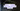# Java - Union and Intersection of two lists

July 04, 2019Suppose you have two lists, and you want Union and Intersection of those two lists.

## Example

``````Input:

list1: [1, 2, 3, 4]
list2: [3, 4, 5, 6]

union(list1, list2): [1, 2, 3, 4, 5, 6]
intersection(list1, list2): [3, 4]``````

## Solution

See the java code for multiple solutions:

``````public static List<Integer> getIntersection_1(List<Integer> l1, List<Integer> l2) {
return l1.stream().filter(l2::contains).collect(Collectors.toList());
}

public static List<Integer> getIntersection_2(List<Integer> l1, List<Integer> l2) {
Set<Integer> s1 = new HashSet<>(l1);
s1.retainAll(l2);
return new ArrayList<>(s1);
}

public static List<Integer> getIntersection_3(List<Integer> l1, List<Integer> l2) {
List<Integer> list = new ArrayList<Integer>();

for (Integer i : l1) {
if(l2.contains(i)) {
}
}

return list;
}

public static List<Integer> getUnion_1(List<Integer> l1, List<Integer> l2) {
Set<Integer> result = new HashSet<Integer>();